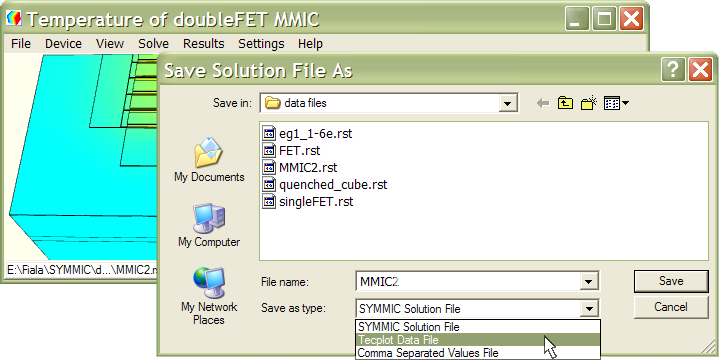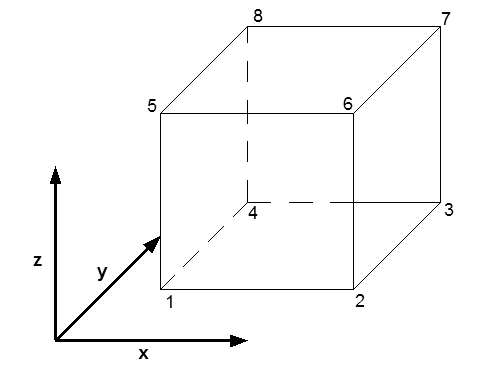SYMMICTM Users Manual
Version 3.1.4

## Saving a Solution

The thermal solution is written to a binary .rst file in the folder that contains the template. For example, the template FET.xml will automatically generate FET.rst as the final solution. This file can be saved using a different filename to preserve the solution for future study, otherwise the file will be over-written by the next thermal simulation involving that template. (Layout simulations wipe out the solution files of the individual devices in the layout.)

Save a solution by selecting Save solution as... from the Solve menu. Choose the file type in the Save as type: list box. Choosing “SYMMIC Solution File” generates a copy of the file in the native binary format. This is the format expected when loading a solution into SYMMIC. Alternatively, the solution file can be saved in an ASCII format that is compatible with Tecplot software, or as a comma separated values file. These text files are generally much larger than the binary format solution files.Solution files in the native binary format are commonly given the .rst file extension, while Tecplot formatted files are usually given the .dat file extension. While this is not strictly required, if a file extension is not included in the filename, the usual one for the format selected will be automatically added.

### Native Binary Format

This format consists of a sequence of binary numbers without any other characters or line terminators. Binary numbers are stored least significant byte first (little endian). Numbers are either 32-bit integers (int32) or 64-bit floating point (double64). Doubles are IEEE-754 standard (1 sign bit, 11-bit exponent, 52-bit significand). Each int32 is 4 bytes, and each double64 is 8 bytes with the last byte having the sign bit. There are no separators between numbers in the file.

The binary file begins with an identifier (always 1), the spatial dimensionality (always 3), and the number N of vertices of the mesh. The (x,y,z) vertices are then listed, resulting in 3N consecutive floating points. The M mesh elements are listed in groups (but usually just 1 group), using the integer index of each vertex to define each mesh element. The first vertex in the file (x1,y1,z1) has index one. Each group of mesh elements is preceded by the number n of vertices per element (usually 8) and the number of elements in the group (usually all M of them). Then the vertices for element 1 are listed in the same order as used for the TecPlot output, followed by the n vertices for element 2, and so on.

Following the mesh elements, there is a sequence of time points (or just one time point for a steady-state solution). Each of the temperature time points begins with an integer index (starting at 1), followed by the time value, and then the temperature value at each of the N vertices listed in order.

The following sequence of binary numbers describes this file format more succinctly.

```int32 1
int32 3
int32 N
double64 x1
double64 y1
double64 z1
double64 x2
double64 y2
double64 z2
:
double64 xN
double64 yN
double64 zN
int32 8
int32 M
int32 e1_v1
int32 e1_v2
int32 e1_v3
int32 e1_v4
int32 e1_v5
int32 e1_v6
int32 e1_v7
int32 e1_v8
int32 e2_v1
int32 e2_v2
:
int32 e2_v8
int32 e3_v1
:
int32 eM_v8
int32 1
double64 time
double64 t1
double64 t2
:
double64 tN
int32 2
double64 time
double64 t1
double64 t2
:
double64 tN
:
int32 T
double64 time
double64 t1
double64 t2
:
double64 tN
```

### Tecplot Format

The Tecplot solution file format consists of lines of ASCII text terminated by newline characters. The file can be read by any text editor. The first line contains the title, the second line contains the variable names, and the third line contains the description of the “zone” of data. Each zone specifies the temperatures over a finite element mesh. The first zone contains the (x,y,z) positions of the mesh nodes and a set of indices describing how these nodes are grouped into finite elements. The third line of the file is the header for the first zone, giving the number of nodes (N) and the number of finite elements (E). The node data begins on the fourth line.

Each of the N node lines is composed of floating point values for the x, y, and z coordinates (in microns) and a temperature value (in kelvin) for a node. The N node lines are followed by E lines containing the element indices. Each element of type FEBRICK is a rectangular brick with 8 corners at the (x,y,z) node points. Each line specifies the node index (integer from 1 to N) of each corner in order shown in the figure.For solution files with only 1 time point (e.g. steady-state solutions), the file does not contain any additional data. But for solutions through time, the first zone may be followed by any number of additional zones containing temperature values for subsequent time points. Each zone begins with a header line that repeats the number of nodes N and finite elements E for the mesh. The zone header also includes a SOLUTIONTIME value that gives the time (in seconds) for this zone. The header line is followed by N lines containing the temperatures for each of the N nodes of the mesh.

The following partial listing demonstrates the format of the Tecplot formatted solution file:

```TITLE = "Tecplot Output"
VARIABLES  = "X" "Y" "Z" "temperature"
ZONE T=" 0.000000000E+000", N=1331, E=1000, ZONETYPE=FEBRICK, DATAPACKING=POINT, SOLUTIONTIME=...
...0.000000000E+000
0.000000000E+000  0.000000000E+000  0.000000000E+000  3.000000000E+002
1.000000000E-002  0.000000000E+000  0.000000000E+000  3.000000000E+002
2.000000000E-002  0.000000000E+000  0.000000000E+000  3.000000000E+002
:                 :                 :                 :
9.000000000E-002  1.000000000E-001  1.000000000E-001  3.000000000E+002
1.000000000E-001  1.000000000E-001  1.000000000E-001  3.000000000E+002
1       2      13      12     122     123     134     133
2       3      14      13     123     124     135     134
3       4      15      14     124     125     136     135
:       :       :       :       :       :       :       :
1197    1198    1209    1208    1318    1319    1330    1329
1198    1199    1210    1209    1319    1320    1331    1330
ZONE T=" 1.000000000E+000", N=1331, E=1000, ZONETYPE=FEBRICK, DATAPACKING=POINT,...
...CONNECTIVITYSHAREZONE=1, VARSHARELIST=([1-3]=1), SOLUTIONTIME= 1.000000000E+000
2.852652045E+002
2.882270777E+002
2.893379757E+002
:
2.882270777E+002
2.852652045E+002
ZONE T=" 2.000000000E+000", N=1331, E=1000, ZONETYPE=FEBRICK, DATAPACKING=POINT,...
...CONNECTIVITYSHAREZONE=1, VARSHARELIST=([1-3]=1), SOLUTIONTIME= 2.000000000E+000
2.778347715E+002
2.813010105E+002
2.831794111E+002
:
2.813010105E+002
2.778347715E+002
ZONE T=" 3.000000000E+000", N=1331, E=1000, ZONETYPE=FEBRICK, DATAPACKING=POINT,...
...CONNECTIVITYSHAREZONE=1, VARSHARELIST=([1-3]=1), SOLUTIONTIME= 3.000000000E+000
:
```

### Comma Separated Values Format

The .csv solution file format consists of lines of ASCII text terminated by newline characters. The file can be read by any text editor or spreadsheet, but many older spreadsheet programs can only load files smaller than 65,536 rows. Most .csv solution files will have many more lines than this.

The first line of the file contains the titles of each column separated by commas. This is followed by the data for each time point in the solution, beginning at time zero. Each data line consists of time, x, y, z, temperature, separated by commas. Each x, y, z triplet represents a node of the mesh. The nodes are listed in ascending order by z, then by y, then x. That is, the x values ascend most rapidly, restarting at each new y value. Nodes can be re-ordered along an axis by sorting rows in the spreadsheet.

The following partial listing demonstrates the format of the .csv solution file:

```time,x,y,z,temperature
0.000000000E+000, 0.000000000E+000, 0.000000000E+000, 0.000000000E+000, 3.000000000E+002
0.000000000E+000, 1.000000000E-002, 0.000000000E+000, 0.000000000E+000, 3.000000000E+002
0.000000000E+000, 2.000000000E-002, 0.000000000E+000, 0.000000000E+000, 3.000000000E+002
0.000000000E+000, 0.000000000E-002, 1.000000000E+000, 0.000000000E+000, 3.000000000E+002
:
```